International
Tables for
Crystallography
Volume A
Space-group symmetry
Edited by M. I. Aroyo

International Tables for Crystallography (2016). Vol. A, ch. 1.6, pp. 107-109

## Section 1.6.2.1. Symmetry information from the diffraction pattern

U. Shmuelia and H. D. Flackb

#### 1.6.2.1. Symmetry information from the diffraction pattern

| top | pdf |

The extraction of symmetry information from the diffraction pattern takes place in three stages.

In the first stage, the unit-cell dimensions are determined and analyzed in order to establish to which Bravais lattice the crystal belongs. A conventional choice of lattice basis (coordinate system) may then be chosen. The determination of the Bravais lattice1 of the crystal is achieved by the process of cell reduction, in which the lattice is first described by a basis leading to a primitive unit cell, and then linear combinations of the unit-cell vectors are taken to reduce the metric tensor (and the cell dimensions) to a standard form. From the relationships amongst the elements of the metric tensor, one obtains the Bravais lattice, together with a conventional choice of the unit cell, with the aid of standard tables. A detailed description of cell reduction is given in Chapter 3.1of this volume and in Part 9of earlier editions (e.g. Burzlaff et al., 2002). An alternative approach (Le Page, 1982) seeks the Bravais lattice directly from the cell dimensions by searching for all the twofold axes present. All these operations are automated in software. Regardless of the technique employed, at the end of the process one obtains an indication of the Bravais lattice and a unit cell in a conventional setting for the crystal system, primitive or centred as appropriate. These are usually good indications which, however, must be confirmed by an examination of the distribution of diffracted intensities as outlined below.

In the second stage, it is the point-group symmetry of the intensities of the Bragg reflections which is determined. We recall that the average reduced intensity of a pair of Friedel opposites (hkl and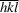) is given by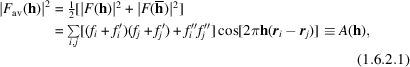where the atomic scattering factor of atom j, taking into account resonant scattering, is given by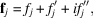the wavelength-dependent components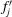and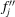being the real and imaginary parts, respectively, of the contribution of atom j to the resonant scattering, h contains in the (row) matrix (1 × 3) the diffraction orders (hkl) and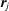contains in the (column) matrix (3 × 1) the coordinates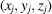of atom j. The components of the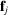are assumed to contain implicitly the displacement parameters. Equation (1.6.2.1)can be found e.g. in Okaya & Pepinsky (1955), Rossmann & Arnold(2001) and Flack & Shmueli (2007). It follows from (1.6.2.1)that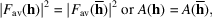regardless of the contribution of resonant scattering. Hence the averaging introduces a centre of symmetry in the (averaged) diffraction pattern.2 In fact, working with the average of Friedel opposites, one may determine the Laue group of the diffraction pattern by comparing the intensities of reflections which should be symmetry equivalent under each of the Laue groups. These are the 11 centrosymmetric point groups:, 2/m, mmm, 4/m, 4/mmm,,, 6/m, 6/mmm,and. For example, the reflections of which the intensities are to be compared for the Laue groupare: hkl, kil, ihl,,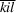and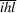, where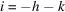. An extensive listing of the indices of symmetry-related reflections in all the point groups, including of course the Laue groups, is given in Appendix 1.4.4 of International Tables for Crystallography Volume B (Shmueli, 2008).3 In the past, one used to inspect the diffraction images to see which classes of reflections are symmetry equivalent within experimental and other uncertainty. Nowadays, the whole intensity data set is analyzed by software. The intensities are merged and averaged under each of the 11 Laue groups in various settings (e.g. 2/m unique axis b and unique axis c) and orientations (e.g.and). For each choice of Laue group and its variant, an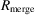factor is calculated as follows: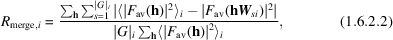where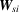is the matrix of theth symmetry operation of the ith Laue group,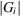is the number of symmetry operations in that group, the average in the first term in the numerator and in the denominator ranges over the intensities of the trial Laue group and the outer summations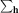range over the hkl reflections. Choices with low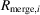display the chosen symmetry, whereas for those with highthe symmetry is inappropriate. The Laue group of highest symmetry with a lowis considered the best indication of the Laue group. Several variants of the above procedure exist in the available software. Whichever of them is used, it is important for the discrimination of the averaging process to choose a strategy of data collection such that the intensities of the greatest possible number of Bragg reflections are measured. In practice, validation of symmetry can often be carried out with a few initial images and the data-collection strategy may be based on this assignment.

In the third stage, the intensities of the Bragg reflections are studied to identify the conditions for systematic absences. Some space groups give rise to zero intensity for certain classes of reflections. These zeros' occur in a systematic manner and are commonly called systematic absences (e.g. in the h0l class of reflections, if all rows with l odd are absent, then the corresponding reflection condition is h0l: l = 2n). In practice, as implemented in software, statistics are produced on the intensity observations of all possible sets of reflections conditions' as given in Chapter 2.3(e.g. in the example above, h0l reflections are separated into sets with l = 2n and those with l = 2n + 1). In one approach, the number of observations in each set having an intensity (I) greater than n standard uncertainties [u(I)] [i.e.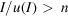] is displayed for various values of n. Clearly, if a trial condition for systematic absence has observations with strong or medium intensity [i.e.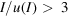], the systematic-absence condition is not fulfilled (i.e. the reflections are not systematically absent). If there are no such observations, the condition for systematic absence may be valid and the statistics for smaller values of n need then to be examined. These are more problematic to evaluate, as the set of reflections under examination may have many weak reflections due to structural effects of the crystal or to perturbations of the measurements by other systematic effects. An alternative approach to examining numbers of observations is to compare the mean value,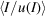, taken over reflections obeying or not a trial reflection condition. For a valid reflection condition, one expects the former value to be considerably larger than the latter. In Section 3.1 of Palatinus & van der Lee (2008), real examples of marginal cases are described.

The third stage continues by noting that the systematic absences are characteristic of the space group of the crystal, although some sets of space groups have identical reflection conditions. In Chapter 2.3one finds all the reflection conditions listed individually for the 230 space groups. For practical use in space-group determination, tables have been set up that present a list of all those space groups that are characterized by a given set of reflection conditions. The tables for all the Bravais lattices and Laue groups are given in Section 1.6.4of this chapter. So, once the reflection conditions have been determined, all compatible space groups can be identified from the tables. Table 1.6.2.1shows that 85 space groups may be unequivocally determined by the procedures defined in this section based on the identification of the Laue group. For other sets of reflection conditions, there are a larger number of compatible space groups, attaining the value of 6 in one case. It is appropriate at this point to anticipate the results presented in Section 1.6.5.1, which exploit the resonant-scattering contribution to the diffracted intensities and under appropriate conditions allow not only the Laue group but also the point group of the crystal to be identified. If such is the case, the last line of Table 1.6.2.1shows that almost all space groups can be unequivocally determined. In the remaining 13 pairs of space groups, constituting 26 space groups in all, there are the 11 enantiomorphic pairs of space groups [(), (), (), (), (), (), (), (), (), () and ()] and the two exceptional pairs of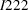&and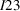&, characterized by having the same symmetry elements in a different arrangement in space. These 13 pairs of space groups cannot be distinguished by the methods described in Sections 1.6.2and 1.6.5.1, but may be distinguished when a reliable atomic structural model of the crystal has been obtained. On the other hand, all these 13 pairs of space groups can be distinguished by the methods described in Section 1.6.6and in detail in Saitoh et al. (2001). It should be pointed out in connection with this third stage that a possible weakness of the analysis of systematic absences for crystals with small unit-cell dimensions is that there may be a small number of axial reflections capable of being systematically absent.

 Table 1.6.2.1| top | pdf | The ability of the procedures described in Sections 1.6.2.1and 1.6.5.1to distinguish between space groups
 The columns of the table show the number of sets of space groups that are indistinguishable by the chosen technique, according to the number of space groups in the set, e.g. for Laue-class discrimination, 85 space groups may be uniquely identified, whereas there are 8 sets containing 5 space groups indistinguishable by this technique. The tables in Section 1.6.4contain 416 different settings of space groups generated from the 230 space-group types.
No. of space groups in set that are indistinguishable by procedure used
123456
No. of sets for Laue-class discrimination 85 78 43 0 8 1
No. of sets for point-group discrimination 390 13 0 0 0 0

It goes without saying that the selected space groups must be compatible with the Bravais lattice determined in stage 1, with the Laue class determined in stage 2 and with the set of space-group absences determined in stage 3.

We thank L. Palatinus (2011) for having drawn our attention to the unexploited potential of the Patterson function for the determination of the space group of the crystal. The discovery of this method is due to Buerger (1946) and later obtained only a one-sentence reference by Rogers (1950) and by Rossmann & Arnold (2001). The method is based on the observation that interatomic vectors between symmetry-related (other than by inversion in a point) atoms cause peaks to accumulate in the corresponding Harker sections and lines of the Patterson function. It is thus only necessary to find the location of those Harker sections and lines that have a high concentration of peaks to identify the corresponding symmetry operations of the space group. At the time of its discovery, it was not considered an economic method of space-group determination due to the labour involved in calculating the Patterson function. Subsequently it was completely neglected and there are no recent reports of its use. It is thus not possible to report on its strengths and weaknesses in practical modern-day applications.

### References

Buerger, M. J. (1946). The interpretation of Harker syntheses. J. Appl. Phys. 17, 579–595.
Burzlaff, H., Zimmermann, H. & de Wolff, P. M. (2002). Crystal lattices. In International Tables for Crystallography, Volume A, Space-Group Symmetry, edited by Th. Hahn, 5th ed., Part 9. Dordrecht: Kluwer Academic Publishers.
Flack, H. D. & Shmueli, U. (2007). The mean-square Friedel intensity difference in P1 with a centrosymmetric substructure. Acta Cryst. A63, 257–265.
Le Page, Y. (1982). The derivation of the axes of the conventional unit cell from the dimensions of the Buerger-reduced cell. J. Appl. Cryst. 15, 255–259.
Okaya, Y. & Pepinsky, R. (1955). Computing Methods and the Phase Problem in X-ray Crystal Analysis, p. 276. Oxford: Pergamon Press.
Palatinus, L. (2011). Private communication.
Palatinus, L. & van der Lee, A. (2008). Symmetry determination following structure solution in P1. J. Appl. Cryst. 41, 975–984.
Rogers, D. (1950). The probability distribution of X-ray intensities. IV. New methods of determining crystal classes and space groups. Acta Cryst. 3, 455–464.
Rossmann, M. G. & Arnold, E. (2001). Patterson and molecular replacement techniques. In International Tables for Crystallography, Volume B, Reciprocal Space, edited by U. Shmueli, ch. 2.3, pp. 235–263. Dordrecht: Kluwer Academic Publishers.
Saitoh, K., Tsuda, K., Terauchi, M. & Tanaka, M. (2001). Distinction between space groups having principal rotation and screw axes, which are combined with twofold rotation axes, using the coherent convergent-beam electron diffraction method. Acta Cryst. A57, 219–230.
Shmueli, U. (2008). Symmetry in reciprocal space. In International Tables for Crystallography, Volume B, Reciprocal Space, edited by U. Shmueli, 3rd ed., ch. 1.4, Appendix A1.4.4. Dordrecht: Springer.